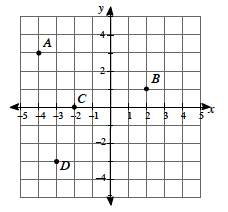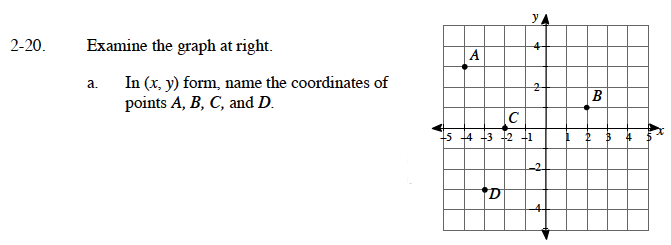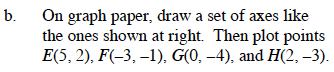### Home > CAAC > Chapter 2 > Lesson 2.1.2 > Problem2-20

2-20.
1. Examine the graph at below. 2-20 HW eTool (Desmos). Homework Help ✎1. In (x, y) form, name the coordinates of points A, B, C, and D.

2. On graph paper, draw a set of axes like the ones shown at right. Then plot points E(5, 2), F(−3, −1), G(0, −4), and H(2, −3).Use the eTool below to input the points into the table and graph the results.
Click the link at right for the full version of the eTool: AC 2-20 HW eTool

Find the change in x and y from the point (0, 0).

Point A goes to the left 4 spaces and up 3 spaces. How do you write that in (x, y) form?

A is (−4, 3). Now find points B, C, and D.